Previous Page | Next Page

 The REG Procedure
 Influence Statistics

This section discusses the INFLUENCE option, which produces several influence statistics, and the PARTIAL option, which produces partial regression leverage plots.

### The INFLUENCE Option

The INFLUENCE option (in the MODEL statement) requests the statistics proposed by Belsley, Kuh, and Welsch (1980) to measure the influence of each observation on the estimates. Influential observations are those that, according to various criteria, appear to have a large influence on the parameter estimates.

Let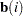be the parameter estimates after deleting theth observation; let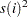be the variance estimate after deleting theth observation; let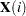be thematrix without theth observation; let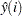be theth value predicted without using theth observation; let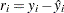be theth residual; and let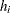be theth diagonal of the projection matrix for the predictor space, also called the hat matrix: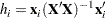Belsley, Kuh, and Welsch (1980) propose a cutoff of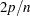, whereis the number of observations used to fit the model andis the number of parameters in the model. Observations withvalues above this cutoff should be investigated.

For each observation, PROC REG first displays the residual, the studentized residual (RSTUDENT), and the. The studentized residual RSTUDENT differs slightly from STUDENT since the error variance is estimated by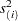without theth observation, not by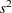. For example,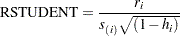Observations with RSTUDENT larger than 2 in absolute value might need some attention.

The COVRATIO statistic measures the change in the determinant of the covariance matrix of the estimates by deleting theth observation: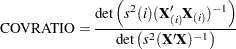Belsley, Kuh, and Welsch (1980) suggest that observations with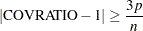whereis the number of parameters in the model andis the number of observations used to fit the model, are worth investigation.

The DFFITS statistic is a scaled measure of the change in the predicted value for theth observation and is calculated by deleting theth observation. A large value indicates that the observation is very influential in its neighborhood of thespace.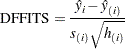Large values of DFFITS indicate influential observations. A general cutoff to consider is 2; a size-adjusted cutoff recommended by Belsley, Kuh, and Welsch (1980) is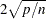, whereandare as defined previously.

The DFFITS statistic is very similar to Cook’s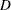, defined in the section Predicted and Residual Values.

The DFBETAS statistics are the scaled measures of the change in each parameter estimate and are calculated by deleting theth observation: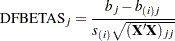where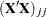is the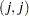th element of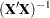.

In general, large values of DFBETAS indicate observations that are influential in estimating a given parameter. Belsley, Kuh, and Welsch (1980) recommend 2 as a general cutoff value to indicate influential observations and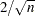as a size-adjusted cutoff.

The following statements use the population example in the section Polynomial Regression. See Figure 74.32 for the fitted regression equation. The INFLUENCE option produces the tables shown in Figure 74.50 and Figure 74.51.

```proc reg data=USPopulation;
model Population=Year YearSq / influence;
run;
```

Figure 74.50 Regression Using the INFLUENCE Option
The REG Procedure
Model: MODEL1
Dependent Variable: Population

Output Statistics
Obs Residual RStudent Hat Diag
H
Cov
Ratio
DFFITS DFBETAS
Intercept Year YearSq
1 -2.2837 -0.9361 0.3429 1.5519 -0.6762 -0.4924 0.4862 -0.4802
2 -0.4146 -0.1540 0.2356 1.5325 -0.0855 -0.0540 0.0531 -0.0523
3 0.6696 0.2379 0.1632 1.3923 0.1050 0.0517 -0.0505 0.0494
4 0.8849 0.3065 0.1180 1.3128 0.1121 0.0335 -0.0322 0.0310
5 0.5923 0.2021 0.0933 1.2883 0.0648 0.0040 -0.0032 0.0025
6 -0.0621 -0.0210 0.0831 1.2827 -0.0063 0.0012 -0.0012 0.0013
7 -0.1344 -0.0455 0.0824 1.2813 -0.0136 0.0054 -0.0055 0.0056
8 0.5864 0.1994 0.0870 1.2796 0.0615 -0.0339 0.0343 -0.0347
9 0.0934 0.0318 0.0933 1.2969 0.0102 -0.0067 0.0067 -0.0068
10 0.2255 0.0771 0.0990 1.3040 0.0255 -0.0182 0.0183 -0.0183
11 1.4757 0.5090 0.1022 1.2550 0.1717 -0.1272 0.1275 -0.1276
12 1.6441 0.5680 0.1022 1.2420 0.1916 -0.1426 0.1426 -0.1424
13 3.4065 1.2109 0.0990 1.0320 0.4013 -0.2895 0.2889 -0.2880
14 1.5922 0.5470 0.0933 1.2345 0.1755 -0.1173 0.1167 -0.1160
15 1.7679 0.6064 0.0870 1.2123 0.1871 -0.1076 0.1067 -0.1056
16 -7.5642 -3.2147 0.0824 0.3286 -0.9636 0.4130 -0.4063 0.3987
17 -7.4712 -3.1550 0.0831 0.3425 -0.9501 0.2131 -0.2048 0.1957
18 -0.3731 -0.1272 0.0933 1.2936 -0.0408 -0.0007 0.0012 -0.0016
19 1.2782 0.4440 0.1180 1.2906 0.1624 0.0415 -0.0432 0.0449
20 1.0356 0.3687 0.1632 1.3741 0.1628 0.0732 -0.0749 0.0766
21 -1.7068 -0.6406 0.2356 1.4380 -0.3557 -0.2107 0.2141 -0.2176
22 4.7578 2.1312 0.3429 0.9113 1.5395 1.0656 -1.0793 1.0933

Figure 74.51 Residual Statistics
 Sum of Residuals -4.7569e-11 170.972 237.712

In Figure 74.50, observations 16, 17, and 19 exceed the cutoff value of 2 for RSTUDENT. None of the observations exceeds the general cutoff of 2 for DFFITS or the DFBETAS, but observations 16, 17, and 19 exceed at least one of the size-adjusted cutoffs for these statistics. Observations 1 and 19 exceed the cutoff for the hat diagonals, and observations 1, 2, 16, 17, and 18 exceed the cutoffs for COVRATIO. Taken together, these statistics indicate that you should look first at observations 16, 17, and 19 and then perhaps investigate the other observations that exceeded a cutoff.

When you enable ODS Graphics, you can request influence diagnostic plots by using the PLOTS= option in the PROC REG statement as shown in the following statements:

```ods graphics on;

proc reg data=USPopulation
plots(label)=(CooksD RStudentByLeverage DFFITS DFBETAS);
id Year;
model Population=Year YearSq;
run;

ods graphics off;
```

The LABEL suboption specified in the PLOTS(LABEL)= option requests that observations that exceed the relevant cutoffs for the statistics being plotted are labeled. Since Year has been named in an ID statement, the value of Year is used for the labels. The requested plots are shown in Figure 74.52.

Figure 74.52 Influence Diagnostics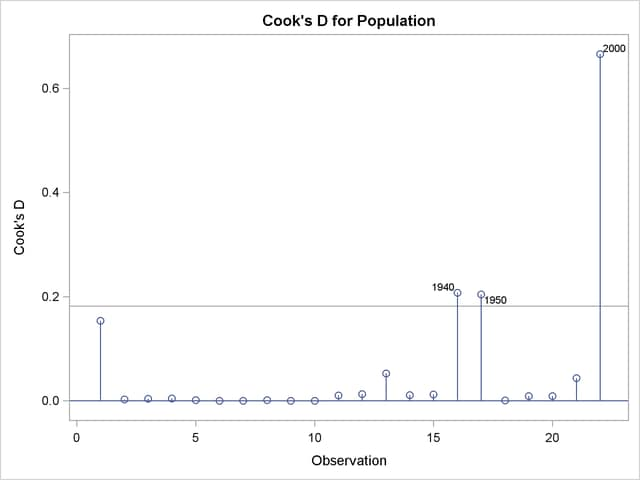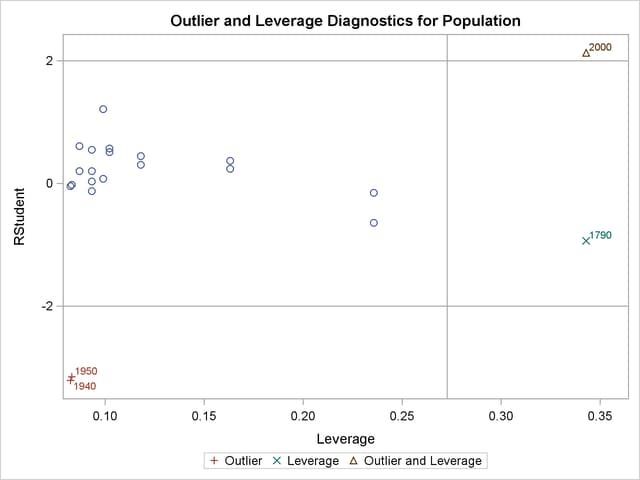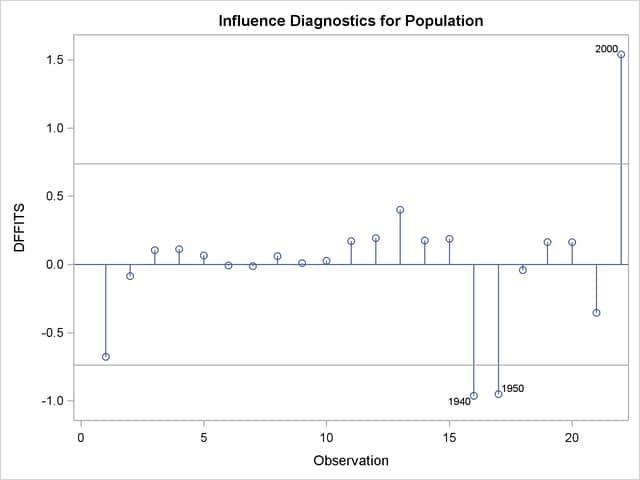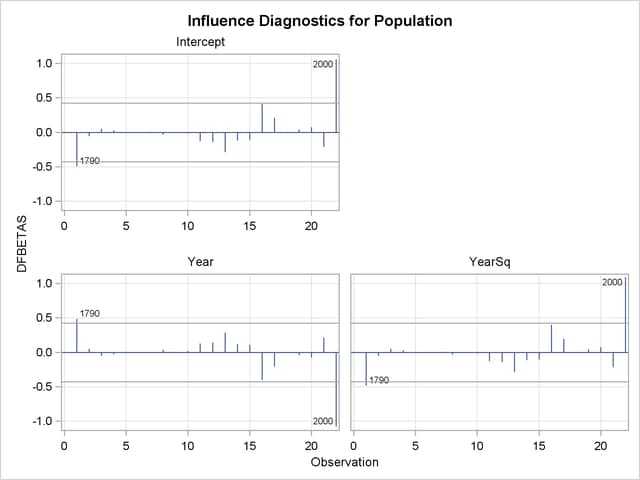### The PARTIAL and PARTIALDATA Options

The PARTIAL option in the MODEL statement produces partial regression leverage plots. If ODS Graphics is not in effect, this option requires the use of the LINEPRINTER option in the PROC REG statement. One plot is created for each regressor in the current full model. For example, plots are produced for regressors included by using ADD statements; plots are not produced for interim models in the various model-selection methods but only for the full model. If you use a model-selection method and the final model contains only a subset of the original regressors, the PARTIAL option still produces plots for all regressors in the full model. If ODS Graphics is in effect, these plots are produced as high-resolution graphics, in panels with a maximum of six partial regression leverage plots per panel. Multiple panels are displayed for models with more than six regressors.

For a given regressor, the partial regression leverage plot is the plot of the dependent variable and the regressor after they have been made orthogonal to the other regressors in the model. These can be obtained by plotting the residuals for the dependent variable against the residuals for the selected regressor, where the residuals for the dependent variable are calculated with the selected regressor omitted, and the residuals for the selected regressor are calculated from a model where the selected regressor is regressed on the remaining regressors. A line fit to the points has a slope equal to the parameter estimate in the full model.

When ODS Graphics is not in effect, points in the plot are marked by the number of replicates appearing at one position. The symbol ’*’ is used if there are 10 or more replicates. If an ID statement is specified, the leftmost nonblank character in the value of the ID variable is used as the plotting symbol.

The PARTIALDATA option in the MODEL statement produces a table that contains the partial regression data that are displayed in the partial regression leverage plots. You can request partial regression data even if you do not requests plots with the PARTIAL option.

The following statements use the fitness data in Example 74.2 with the PARTIAL option and the ODS GRAPHICS statement to produce the partial regression leverage plots. The plots are shown in Figure 74.53.

```ods graphics on;

proc reg data=fitness;
model Oxygen=RunTime Weight Age / partial;
run;

ods graphics off;
```

Figure 74.53 Partial Regression Leverage Plots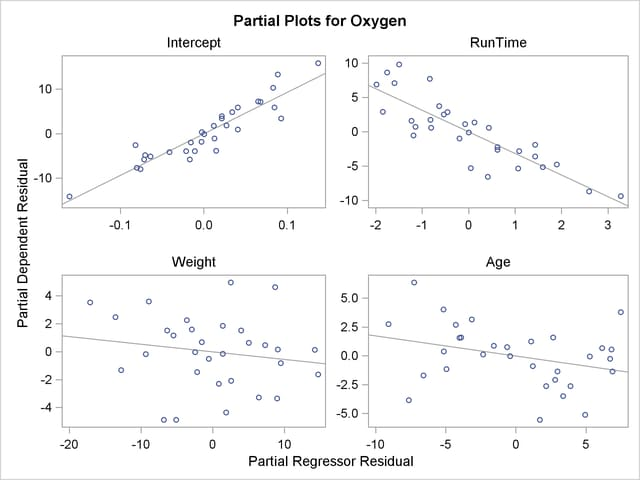Previous Page | Next Page | Top of Page